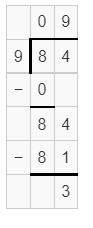# McGraw Hill Math Grade 4 Chapter 6 Lesson 2 Answer Key Dividing a Two-Digit Number with Remainders

Practice the questions of McGraw Hill Math Grade 4 Answer Key PDF Chapter 6 Lesson 2 Dividing a Two-Digit Number with Remainders to secure good marks & knowledge in the exams.

## McGraw-Hill Math Grade 4 Answer Key Chapter 6 Lesson 2 Dividing a Two-Digit Number with Remainders

Divide

Find each quotient and remainder.

Question 1.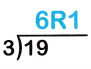Explanation:
quotient = 6
remainder = 1

Question 2.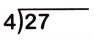Explanation:
quotient = 6
remainder = 3

Question 3.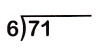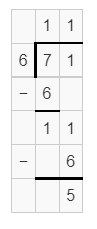Explanation:
quotient = 11
remainder = 5

Question 4.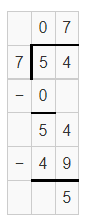Explanation:
quotient = 7
remainder = 5

Question 5.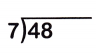Explanation:
quotient = 6
remainder = 6

Question 6.Explanation:
quotient = 40
remainder = 1

Question 7.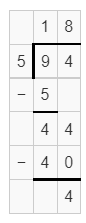Explanation:
quotient = 18
remainder = 4

Question 8.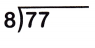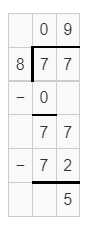Explanation:
quotient = 9
remainder = 5

Question 9.
Explain why 84 ÷ 5 has two digits in the quotient while 84 ÷ 9 has only one digit in the quotient.